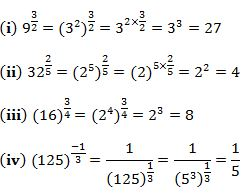# NCERT Solutions for Class 9th: Ch 1 Number Systems Maths

#### NCERT Solutions for Class 9th: Ch 1 Number Systems Maths

Page No: 5

Exercise 1.1

1. Is zero a rational number? Can you write it in the form p/q, where p and q are integers and q ≠ 0?

Yes. Zero is a rational number as it can be represented as 0/1 or 0/2.

2. Find six rational numbers between 3 and 4.

There are infinite rational numbers in between 3 and 4.
3 and 4 can be represented as 24/8 and 32/8 respectively.
Therefore, six rational numbers between 3 and 4 are
25/8, 26/8, 27/8, 28/8, 29/8, 30/8.

3. Find five rational numbers between 3/5 and 4/5.

There are infinite rational numbers in between 3/5 and 4/5
3/5 = 3×6/5×6 = 18/30
4/5 = 4×6/5×6 = 24/30
Therefore, five rational numbers between 3/5 and 4/5 are
19/30, 20/30, 21/30, 22/30, 23/30.

4. State whether the following statements are true or false. Give reasons for your answers.

(i) Every natural number is a whole number.
► True, since the collection of whole numbers contains all natural numbers.

(ii) Every integer is a whole number.
► False, as integers may be negative but whole numbers are always positive.

(iii) Every rational number is a whole number.
► False, as rational numbers may be fractional but whole numbers may not be.

Page No: 8

Exercise 1.2

1. State whether the following statements are true or false. Justify your answers.

(i) Every irrational number is a real number.
► True, since the collection of real numbers is made up of rational and irrational numbers.

(ii) Every point on the number line is of the form√m, where m is a natural number.
► False, since positive number cannot be expressed as square roots.

(iii) Every real number is an irrational number.
► False, as real numbers include both rational and irrational numbers. Therefore, every real number cannot be an irrational number.

2. Are the square roots of all positive integers irrational? If not, give an example of the square root of a number that is a rational number.

No, the square roots of all positive integers are not irrational. For example √4 = 2.

3. Show how √5 can be represented on the number line.

Step 1: Let AB be a line of length 2 unit on number line.
Step 2: At B, draw a perpendicular line BC of length 1 unit. Join CA.
Step 3: Now, ABC is a right angled triangle. Applying Pythagoras theorem,
AB2 + BC2 = CA2
⇒ 22 + 12 = CA2
⇒ CA2 = 5
⇒ CA = √5
Thus, CA is a line of length √5 unit.
Step 4: Taking CA as a radius and A as a centre draw an arc touching
the number line. The point at which number line get intersected by
arc is at √5 distance from 0 because it is a radius of the circle
whose centre was A.
Thus, √5 is represented on the number line as shown in the figure.Page No: 14

Exercise 1.3

1. Write the following in decimal form and say what kind of decimal expansion each has:
(i) 36/100
= 0.36 (Terminating)

(ii) 1/11
0.09090909... = 0.9 (Non terminating repeating)

= 33/8 = 4.125 (Terminating)

(iv) 3/13
= 0.230769230769... = 0.230769 (Non terminating repeating)

(v) 2/11
= 0.181818181818... = 0.18 (Non terminating repeating)

(vi) 329/400
= 0.8225 (Terminating)

2. You know that 1/7 = 0.142857.Can you predict what the decimal expansion of 2/7, 3/7, 4/7, 5/7, 6/7 are without actually doing the long division? If so, how?
[Hint: Study the remainders while finding the value of 1/7 carefully.]

Yes. We can be done this by: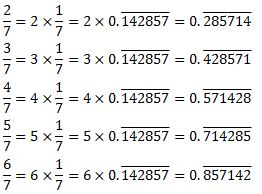3. Express the following in the form p/q where p and q are integers and q ≠ 0.
(i) 0.6
(ii) 0.47
(iii) 0.001

(i) 0.6 = 0.666...
Let x = 0.666...
10x = 6.666...
10x = 6 + x
9x = 6
x = 2/3

(ii) 0.47 = 0.4777...
= 4/10 + 0.777/10
Let x = 0.777…
10x = 7.777…
10x = 7 + x
x = 7/9
4/10 + 0.777.../10 = 4/10 + 7/90
= 36/90 +7/90 = 43/90

(iii) 0.001 = 0.001001...
Let x = 0.001001...
1000x = 1.001001…
1000x = 1 + x
999x = 1
x = 1/999

4. Express 0.99999…in the form  p/q. Are you surprised by your answer? With your teacher and classmates discuss why the answer makes sense.

Let x = 0.9999…
10x = 9.9999…
10x = 9 + x
9x = 9
x = 1
The difference between 1 and 0.999999 is 0.000001 which is negligible. Thus, 0.999 is too much near 1, Therefore, the 1 as answer can be justified.

5. What can the maximum number of digits be in the repeating block of digits in the decimal expansion of 1/17? Perform the division to check your answer.

1/17 = 0.0588235294117647
There are 16 digits in the repeating block of the decimal expansion of 1/17.
Division Check: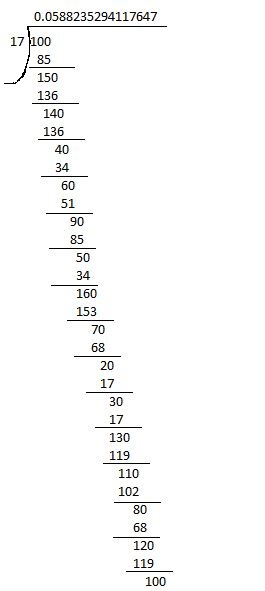= 0.0588235294117647

6. Look at several examples of rational numbers in the form p/(≠ 0) where p and q are integers with no common factors other than 1 and having terminating decimal representations (expansions). Can you guess what property q must satisfy?

We observe that when q is 2, 4, 5, 8, 10... then the decimal expansion is terminating. For example:
1/2 = 0.5, denominator q = 21
7/8 = 0.875, denominator q = 23
4/5 = 0.8, denominator q = 51

We can observed that terminating decimal may be obtained in the situation where prime factorisation of the denominator of the given fractions has the power of 2 only or 5 only or both.

7. Write three numbers whose decimal expansions are non-terminating non-recurring.

Three numbers whose decimal expansions are non-terminating non-recurring are:
0.303003000300003...
0.505005000500005...
0.7207200720007200007200000…

8. Find three different irrational numbers between the rational numbers 5/7 and 9/11.

5/7 = 0.714285
9/11 = 0.81
Three different irrational numbers are:
0.73073007300073000073…
0.75075007300075000075…
0.76076007600076000076…

9. Classify the following numbers as rational or irrational:
(i) √23
(ii) √225
(iii) 0.3796
(iv) 7.478478
(v) 1.101001000100001…

(i) √23 = 4.79583152331...
Since the number is non-terminating non-recurring therefore, it is an irrational number.

(ii) √225 = 15 = 15/1
Since the number is rational number as it can represented in p/form.

(iii) 0.3796
Since the number is terminating therefore, it is an rational number.

(iv) 7.478478 = 7.478
Since the this number is non-terminating recurring, therefore, it is a rational number.

(v) 1.101001000100001…
Since the number is non-terminating non-repeating, therefore, it is an irrational number.

Page No: 18

Exercises 1.4

1. Visualise 3.765 on the number line using successive magnification.2. Visualise 4.26 on the number line, up to 4 decimal places.

4.26 = 4.2626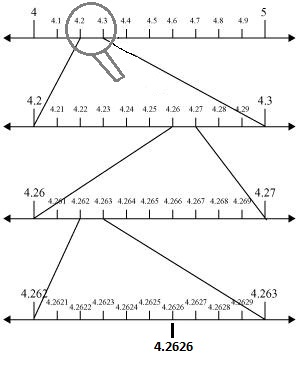Page No: 24

Exercise 1.5

1. Classify the following numbers as rational or irrational:
(i) 2 - √5
(ii) (3 + √23) - √23
(iii) 2√7/7√7
(iv) 1/2
(v) 2π

(i) 2 - √5 = 2 - 2.2360679… = - 0.2360679…
Since the number is is non-terminating non-recurring therefore, it is an irrational number.

(ii) (3 + √23) - √23 = 3 + √23 - √23 = 3 = 3/1
Since the number is rational number as it can represented in p/form.

(iii) 2√7/7√7 = 2/7
Since the number is rational number as it can represented in p/form.

(iv) 1/2 = √2/2 = 0.7071067811...
Since the number is is non-terminating non-recurring therefore, it is an irrational number.

(v) 2π = 2 × 3.1415… = 6.2830…
Since the number is is non-terminating non-recurring therefore, it is an irrational number.

2. Simplify each of the following expressions:

(i) (3 + √3) (2 + √2)
(ii) (3 + √3) (3 - √3)
(iii) (√5 + √2)2
(iv) (√5 - √2) (√5 + √2)

(i) (3 + √3) (2 + √2)
⇒ 3 × 2 + 2 + √3 + 3√2+ √3 ×√2
⇒ 6 + 2√3 +3√2 + √6

(ii) (3 + √3) (3 - √3) [∵ (a + b) (a - b) = a2 - b2]
⇒ 32 - (√3)2
⇒ 9 - 3
⇒ 6

(iii) (√5 + √2)[∵ (a + b)2 = a2 + b2 + 2ab]
⇒ (√5)2 + (√2)2 + 2 ×√5 × √2
⇒ 5 + 2 + 2 × √5× 2
⇒ 7 +2√10

(iv) (√5 - √2) (√5 + √2) [∵ (a + b) (a - b) = a2 - b2]
⇒ (√5)2 - (√2)2
⇒ 5 - 2
⇒ 3

3. Recall, π is defined as the ratio of the circumference (say c) of a circle to its diameter (say d). That is, π = c/d. This seems to contradict the fact that π is irrational. How will you resolve this contradiction?

There is no contradiction. When we measure a value with a scale, we only obtain an approximate value. We never obtain an exact value. Therefore, we may not realise that either c or d is irrational. The value of π is almost equal to 22/7 or 3.142857...

4. Represent √9.3 on the number line.

Step 1: Draw a line segment of unit 9.3. Extend it to C so that BC is of 1 unit.
Step 2: Now, AC = 10.3 units. Find the centre of AC and name it as O.
Step 3: Draw a semi circle with radius OC and centre O.
Step 4: Draw a perpendicular line BD to AC at point B which intersect the semicircle at D. Also, Join OD.
Step 5: Now, OBD is a right angled triangle.
Here, OD = 10.3/2 (radius of semi circle), OC = 10.3/2, BC = 1
OB = OC – BC = (10.3/2) – 1 = 8.3/2
Using Pythagoras theorem,
OD2 = BD2 + OB2
⇒ (10.3/2)2 = BD2 + (8.3/2)2
⇒ BD2 = (10.3/2)2 - (8.3/2)2
⇒ BD2 = (10.3/2 – 8.3/2) (10.3/2 + 8.3/2)
⇒ BD2 = 9.3
⇒ BD2 =  √9.3
Thus, the length of BD is √9.3.
Step 6: Taking BD as radius and B as centre draw an arc which touches the line segment. The point where it touches the line segment is at a distance of √9.3 from O as shown in the figure.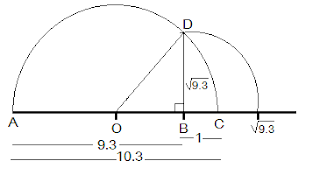5. Rationalise the denominators of the following:
(i) 1/7
(ii) 1/7-√6
(iii) 1/5+√2
(iv) 1/7-2Page No: 26

Exercise 1.6

1. Find:
(i) 641/2
(ii) 321/5
(iii) 1251/32. Find:
(i) 93/2
(ii) 322/5
(iii) 163/4
(iv) 125-1/3